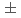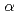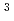We have measured neutron flux distribution around the core tank of STACY heterogeneous core by position sensitive proportional counter (PSPC) to develop the method to measure reactivity for subcritical systems. The neutron flux distribution data in the position accuracy of13mm have been obtained in the range of uranium concentration of 50g/L to 210g/L both in critical and in subcritical state. The prompt neutron decay constant,, was evaluated from the measurement data of pulsed neutron source experiments. We also calculated distribution of neutron flux andHe reaction rates at the location of PSPC by using continuous energy Monte Carlo code MCNP. The measurement data was compared with the calculation results. As results of comparison, calculated values agreed generally with measurement data of PSPC with Cd cover in the region above half of solution height, but the difference between calculated value and measurement data was large in the region below half of solution height. On the other hand, calculated value agreed well with measurement data of PSPC without Cd cover.